Download PDF文章快速检索 高级检索

Space interception orbit optimization design based on hybrid optimal algorithm
GAO Xiaoguang, TANG Hong, DUAN Junhong
College of Electronic and Information, Northwestern Polytechnical University, Xi'an 710072, China
Abstract: Based on a hybrid algorithm combining genetic algorithm (GA) with improved Gauss method (IGM), a design method of space interception orbit was proposed for solving time-fuel-optimal trajectory planning problem of interceptor. First, classical Gauss method was improved by applying Newton-Raphson iteration, solving the problem of the classical Gauss method of slow convergence speed and small transfer angle. Then, a theorem on the necessary and sufficient condition for the existence of unique solution was proved. When the initial orbital parameters were given, this condition could be used to judge whether elliptical orbit could be introduced as the interception orbit. After that, constraints of transfer time and maximum pulse rate were given, as well as the calculation steps of hybrid optimal algorithm, and way of coding was improved. Finally taking optimization problem of space interception orbit as an example, simulation was carried out. Simulation result shows that the hybrid algorithm has fewer generations and shorter consuming time compared with conventional optimal algorithm, indicating the algorithm is applicable in determining interception orbit in space.
Key words: optimization     space interception     Gauss method     genetic algorithm (GA)     hybrid algorithm

1 空间拦截问题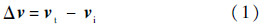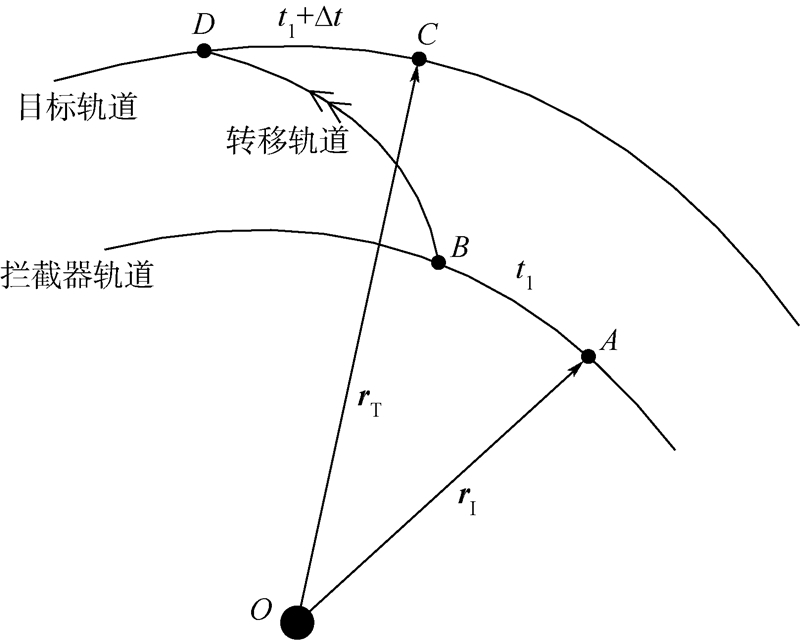图 1 空间拦截问题示意图 Fig. 1 Schematic diagram of space interception
2 改进高斯法

1) 当转移角大于π/2时,方法失效.

2) 原始高斯法的迭代初值可能不在函数迭代收敛域内.

3) 函数迭代收敛时,线性收敛速度慢.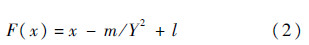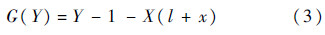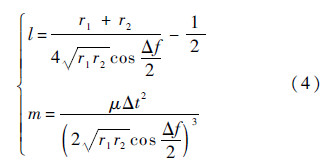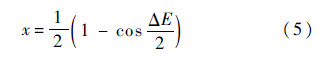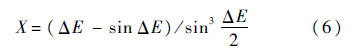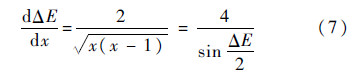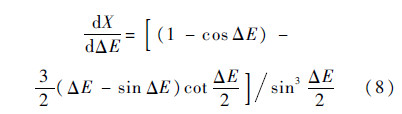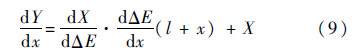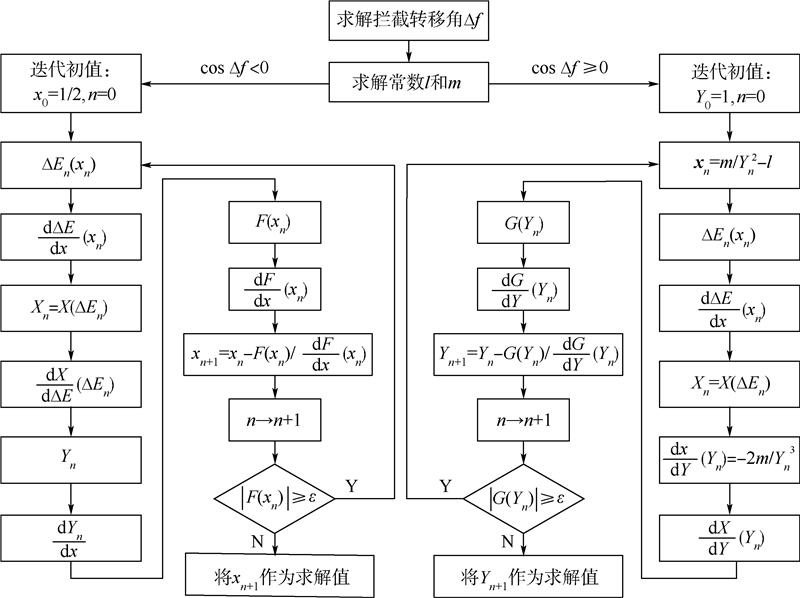图 2 改进高斯法流程图 Fig. 2 low chart of IGM

 比较参数 原始高斯法 改进高斯法 求解时间/ms 54.4 44.8

3 迭代方程有唯一解的充要条件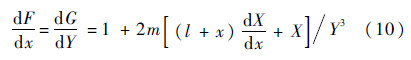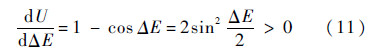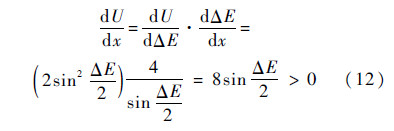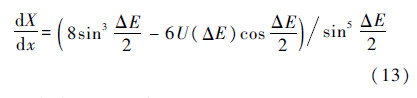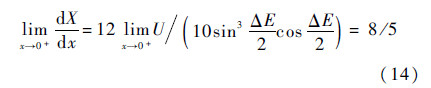x∈[1/2,1)时,由式(13)可知dXdx>0.

x∈［0,1/2],ΔE∈[0,π]时,令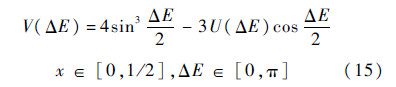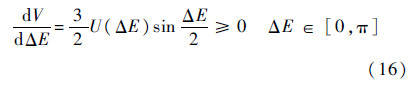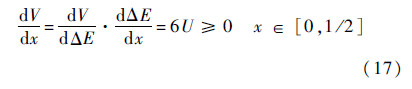X(0)=4/3和dXdx≥0,可知X(x)≥4/3,x∈[0,1).经过前面分析可知,F(x)G(Y)分别在它们的定义域上单调增.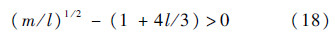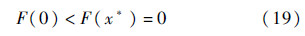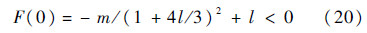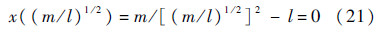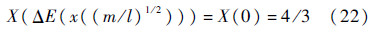G(Y)的单调性可知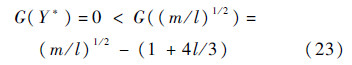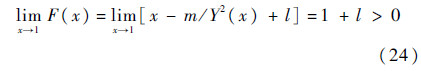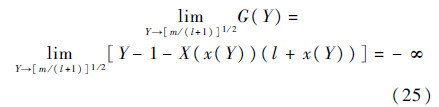4.1 约束条件

1) 开始转移时刻应小于目标轨道周期的1/2,即tk<1/2tp,拦截时刻应小于目标轨道周期,即te<tp,从而使目标航天器预警机动的时间减少,提高命中概率.

2) 拦截器变轨是在一次速度脉冲的作用下瞬间完成的.轨道转移能量消耗应有上限约束,即Δv≤vmax,本文将拦截器最大变轨脉冲限制为vmax=8.5km/s.

4.2 变量的编码

4.3 适应度函数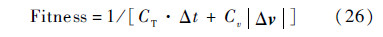4.4 混合优化算法设计步骤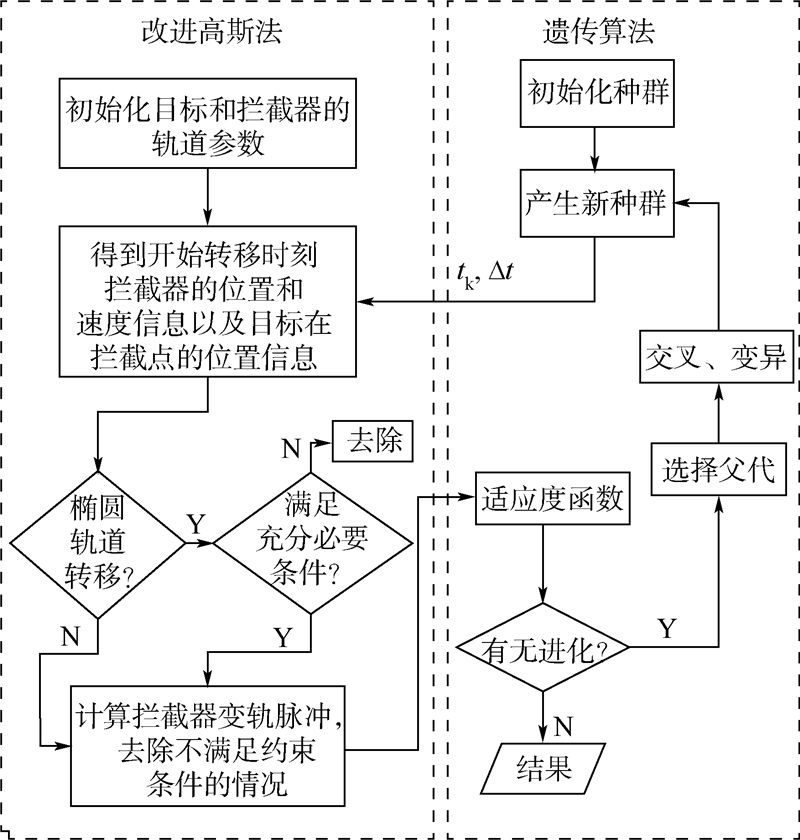图 3 混合优化算法流程图 Fig. 3 Flow chart of hybrid optimal algorithm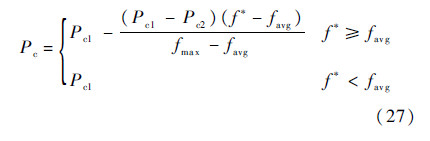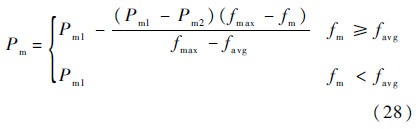5 仿真算例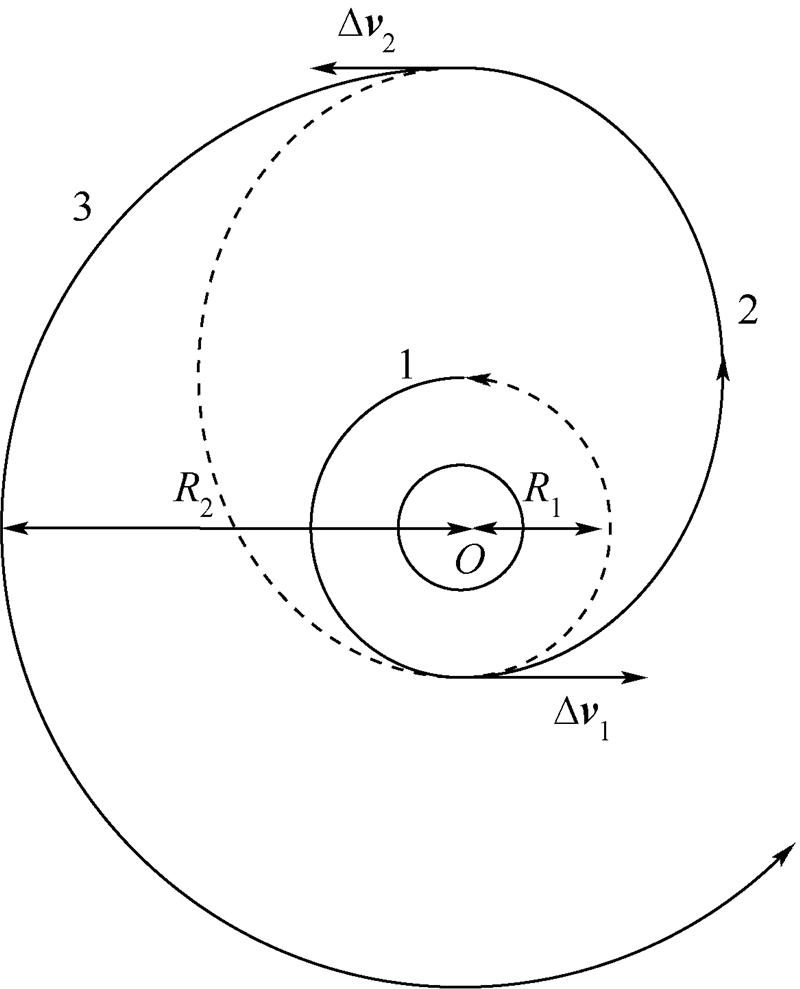图 4 霍曼转移示意图 Fig. 4 Schematic diagram of Homan transfer

 算法 Δt/s Δf/(°) Δv/(km·s-1) 霍曼转移 6136.6 180.0 1.0029 文献算法 6102.8 178.9 0.9961 本文算法 6124.8 179.8 1.00291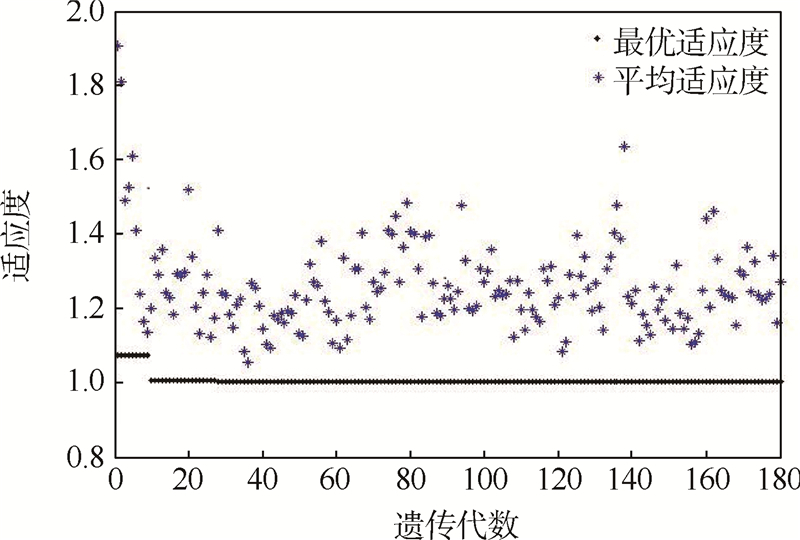图 5 适应度值随遗传代数的变化 Fig. 5 Variation of fitness value via generation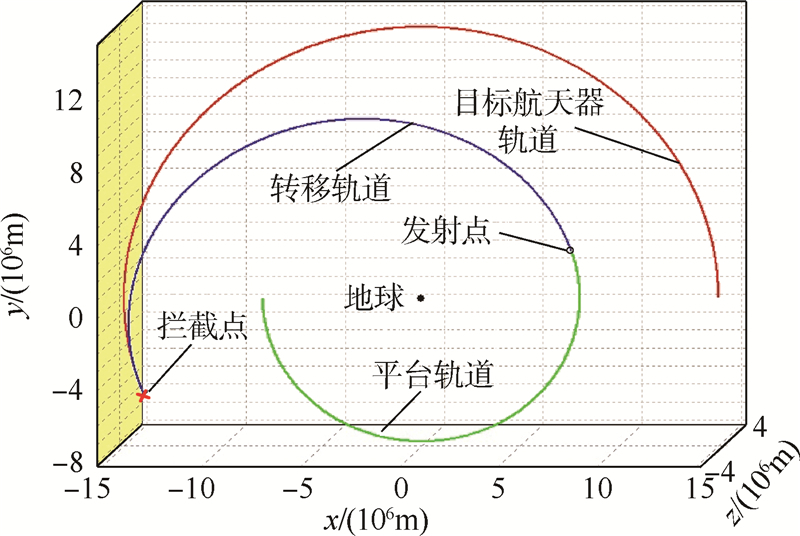图 6 共面圆间转移的运动轨迹 Fig. 6 Trajectory of coplanar transfer

 组别 权重因子 Δt/s |Δf|/(°) Δv/(km·s－1) e 1 CT=0,Cv=1 13697.1 241.3 1.4853 0.465 2 CT=0.3,Cv=0.7 4272.9 112.4 2.5007 0.806 3 CT=1,Cv=0 2685.4 24.3 8.1571 0.958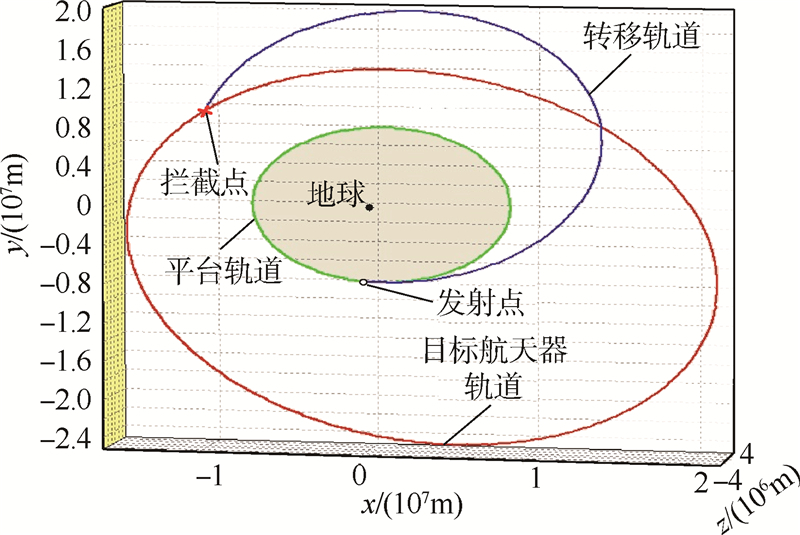图 7 CT=0,Cv=1时目标器与拦截器的运动轨迹 Fig. 7 Trajectory of target and interceptor when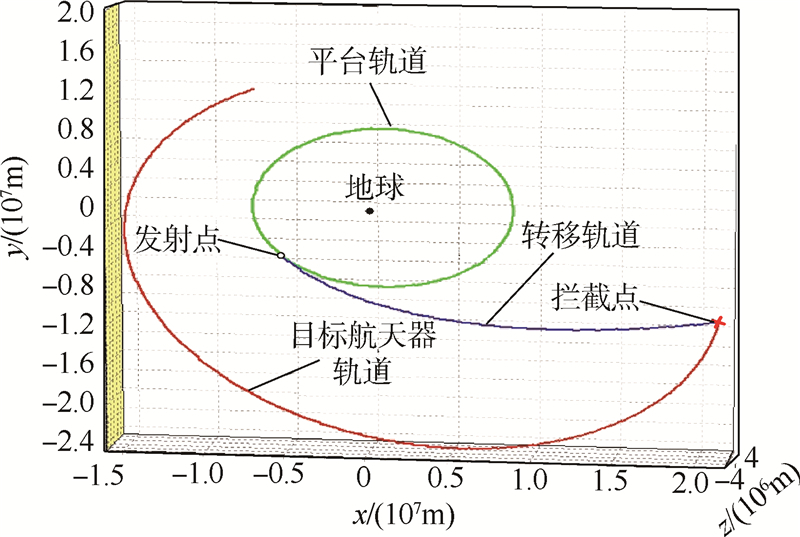图 8 CT=0.3,Cv=0.7时目标器与拦截器的运动轨迹 Fig. 8 Trajectory of target and interceptor when CT=0.3,Cv=0.7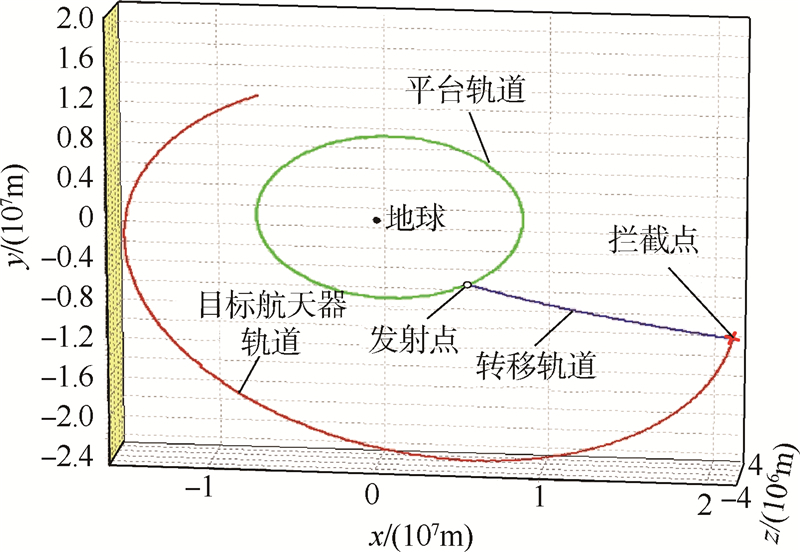图 9 CT=1,Cv=0时目标器与拦截器的运动轨迹 Fig. 9 Trajectory of target and interceptor when CT=1,Cv=0

6 结 论

1) 此方法不需要初始猜测,避免了传统轨道优化方法中初始猜测的困难.

2) 可以求得全局最优解,克服了某些传统优化方法容易收敛于局部最优解的困难.

3) 与传统优化方法相比,收敛精度和收敛速度有所提高.

  晁涛, 王松艳, 王子才, 等.基于组合优化算法的临近空间飞行器轨迹优化[J].宇航学报, 2012, 33(2):183-188. Chao T, Wang S Y, Wang Z C, et al.Near space vehicle trajectory optimization approach based on hybrid SVM and GA algorithm[J].Journal of Astronautics, 2012, 33(2):183-188(in Chinese). Cited By in Cnki  Shen H J, Tsiotras P.Optimal two-impulse rendezvous using multiple-revolution Lambert solutions[J].Journal of Guidance, Control, and Dynamics, 2003, 26(1):50-61. Click to display the text  周荻, 张刚, 孙胜.有限推力椭圆轨道近距离拦截方法[J].宇航学报, 2010, 31(7):1763-1767. Zhou D, Zhang G, Sun S.A thrust-limited short-distance interception scheme on elliptical orbit[J].Journal of Astronautics, 2010, 31(7):1763-1767(in Chinese). Cited By in Cnki (2)  Spencer D B, Kim Y H.Optimal spacecraft rendezvous using genetic algorithms[J].Journal of Spacecraft and Rockets, 2002, 39(6):859-865. Click to display the text  黄勇, 李小将, 张东来, 等.混合遗传算法在最优Lambert轨道转移设计中的应用[J].飞行力学, 2013, 31(3):269-272. Huang Y, Li X J, Zhang D L, et al.Application of hybrid genetic algorithm in optimal Lambert orbital transfer design[J].Flight Dynamics, 2013, 31(3):269-272(in Chinese). Cited By in Cnki (1)  Kim D Y, Woo B, Park S Y, et al.Hybrid optimization for multiple-impulse reconfiguration trajectories of satellite formation flying[J].Advances in Space Research, 2009, 44(1):1257-1269. Click to display the text  Battin R H.An introduction to the mathematics and methods of astrodynamics[M].Revised Edition.New York:AIAA, 1999. Click to display the text  邓泓, 仲惟超, 孙兆伟, 等.基于遗传算法的卫星攻击路径规划方法研究[J].宇航学报, 2009, 30(4):1587-1592. Deng H, Zhong W C, Sun Z W, et al.Method research of satellite attacking path planning based on genetic algorithm[J].Journal of Astronautics, 2009, 30(4):1587-1592(in Chinese). Cited By in Cnki (2)  陈统, 徐世杰.基于遗传算法的最优Lambert双脉冲转移[J].北京航空航天大学学报, 2007, 33(3):273-277. Chen T, Xu S J.Optimal Lambert two-impulse transfer using genetic algorithm[J].Journal of Beijing University of Aeronautics and Astronautics, 2007, 33(3):273-277(in Chinese). Cited By in Cnki (37)  高怀, 朱战霞, 刘剑.基于遗传算法的连续推力最短时间转移轨道设计[J].西北工业大学学报, 2012, 30(2):187-191. Gao H, Zhu Z X, Liu J.An effective minimum-time orbital transfer design under continuous thrust[J].Journal of Northwestern Polytechnical University, 2012, 30(2):187-191(in Chinese). Cited By in Cnki  Danchick R.Gauss meets newton again: How to make Gauss orbit determination from two position vectors more efficient and robust with Newton-Raphson iterations[J].Applied Mathematics and Computation, 2008, 195(2):364-375. Click to display the text  Abdelkhalik O, Mortari D.N-impulse orbit transfer using genetic algorithms[J].Journal of Spacecraft and Rockets, 2007, 44(2):456-460. Click to display the text  齐映红, 曹喜滨.基于遗传算法的最优多脉冲交会轨道设计[J].哈尔滨工业大学学报, 2008, 40(9):1345-1348. Qi Y H, Cao X B.Design of multiple-impulsive rendezvous trajectory using genetic algorithms[J].Journal of Harbin Institute of Technology, 2008, 40(9):1345-1348(in Chinese). Cited By in Cnki (7)  王威, 于志坚.航天器轨道确定-模型与算法[M].北京:国防工业出版社, 2007:76-87. Wang W, Yu Z J.Orbit determination-modeling and algorithm[M].Beijing:Defense Industry Press, 2007:76-87(in Chinese).  Srinivas M, Patnaik L M.Adaptive probabilities of crossover and mutation in genetic algorithm[J].IEEE Transaction on Systems, Man and Cybernetics, 1994, 24(4):656-666. Click to display the text

#### 文章信息

GAO Xiaoguang, TANG Hong, DUAN Junhong

Space interception orbit optimization design based on hybrid optimal algorithm

Journal of Beijing University of Aeronautics and Astronsutics, 2015, 41(9): 1574-1581.
http://dx.doi.org/10.13700/j.bh.1001-5965.2014.0673